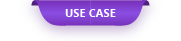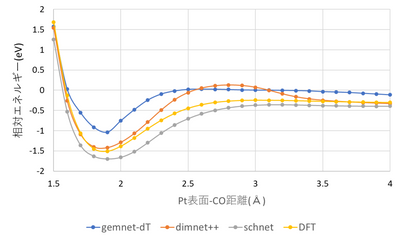A molecular dynamics simulation of the catalytic material was performed using a general-purpose graph neural network force field (GNN force field).

# Molecular dynamics simulation of catalytic materials using general-purpose graph neural network force fields

A molecular dynamics simulation of the catalytic material was performed using a general-purpose graph neural network force field (GNN force field). The GNN force field used was developed by the Open Catalyst Project  sponsored by Facebook and Carnegie Mellon University, and is the calculation result of about 130 million first-principles calculations targeting catalytic materials. Is constructed as training data, and molecular dynamics calculations of a wide range of catalytic materials are possible. In this case, the adsorption potential curve of CO molecules on the platinum catalyst surface was calculated using the GNN force field, and the accuracy of the GNN force field was confirmed by comparing it with the first-principles calculation results.

#### Calculation model / calculation conditions

The adsorption of CO molecules on the surface of the platinum catalyst was calculated using the calculation model shown in Fig. 1. Table 1 also shows the first-principles calculation conditions and the GNN force field method used.

First-principles calculation conditionsGNN calculation conditionsCode usedVASP (ver 5.4.4)Code usedLAMMPS Exchange correlation functionalRPBEUse GNNGemnet + dTPseudopotentialPAWDimnet ++Cutoff energy400eVSchnetk-point sampling3 × 3 × 1

#### Calculation result

Figure 2 shows the calculation results of the adsorption potential curve, and Table 2 shows the calculation results of the adsorption energy. Although there are differences in the calculation results depending on the GNN force field method used, it can be seen that the adsorption energy can be predicted with an accuracy of 0.5 eV or less. Comparing the time required to calculate the energy per point, it was confirmed that the GNN force field can be calculated 200 times faster than the first-principles calculation.

MethodAdsorption energy (eV)Calculation time per point (sec) *Gemnet-dT-1.03715Dimnet ++-1.42015Schnet-1.69615DFT-1.5104000

Table 2 Adsorption energy calculation results (* GNN force field is one GPU (V100), DFT is calculation time per CPU core)

#### Summary

• By using a general-purpose GNN force field, it is possible to calculate catalyst materials at low cost and at high speed with the same calculation accuracy as first-principles calculation.
• Since it is a force field constructed using a huge amount of learning data, it is possible to simulate a wide range of catalytic materials.

 Chanussot Lowik et.al. "Open Catalyst 2020 (OC20) Dataset and Community Challenges", ACS Catalysis (2020)

 Customized LAMMPS (7Aug2019) for Neural Network Potential, by AdvanceSoft Corp. http://www.advancesoft.jp.

Original Source from: https://ctc-mi-solution.com/汎用型グラフニューラルネットワーク力場を用い/